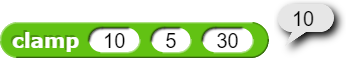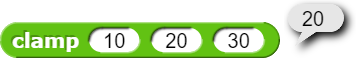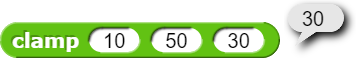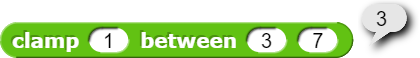# Clamp function

Let’s just cut to the chase… I have literally no idea where to start with clamp… clamp() - CSS: Cascading Style Sheets | MDN this is the function I’m referring to and I have zero clue as to how to implement it

I did, and that is what the block does.Basically it’s the map nodon from GBG, one of the most useful functions from that game and I really can’t seem to find an equivalent anywhere

“outputs a value mapped from its Input Range on to its Output Range”
-Nintendo

For example
Clamp (1) between (3) and (7)
Would give 3

It also exists inside of blender out of all things

like this?Maybe, what’s the definition?

They said it earlier on.

yup, all I did was rearrange the inputs.

Yeah that isn’t it, there’s no mathematical equivalent to it that I can find making it difficult to explain

It would help if you gave us 3 examples, when the value is less than the minimum, when the value is inside the range, and when the value is above the maximum.

The clamp function you're describing can be written as the following, correct?

If a value is less than a minimum, return the minimum.
If the value is greater than a maximum, return the maximum.
Otherwise, return the value.

Ego-lay's block does that: it returns the larger out of the minimum and the value and then the smaller of that and the maximum.

No it’s not a constrain function, it’s mapping between 2 values

Do you want something like this?

Ya that’s exactly what I need but with only 1 axis

FIgure out how wide your input domain is. (Max minus min.)

Take the input value you're interested in and subtract the min of the domain. Divide by the width, and you have a number between 0 and 1 that tells you what fraction of the way your value is.

Figure out how wide the output range is. (f(max) minus f(min))

Now take your fraction of the width of the range, add f(min), and you're all set.

Is that what you need?

This?

Yeah I can see why I had no clue where to start

The block you’re proposing has three terms. The first and third term are equal, and annihilate each other. So effectively only the second term is used. It boils down to:

clamp (value, minimum, maximum) = minimum · (value - minimum) / (maximum - minimum)

… and I don’t have a clue as to what that represents.

Long story short: @ego-lay_atman-bay’s formula is correct, unless min > max.